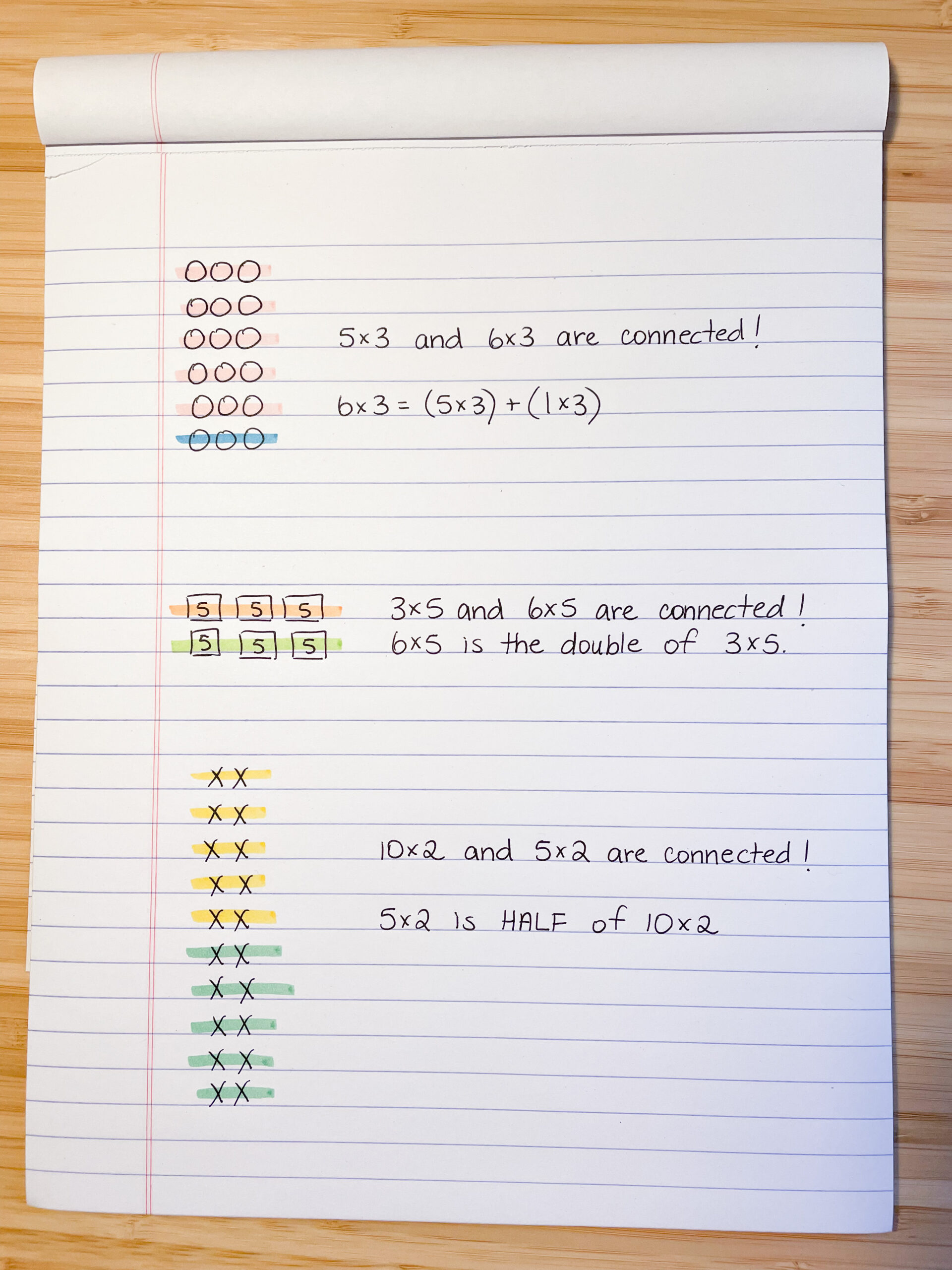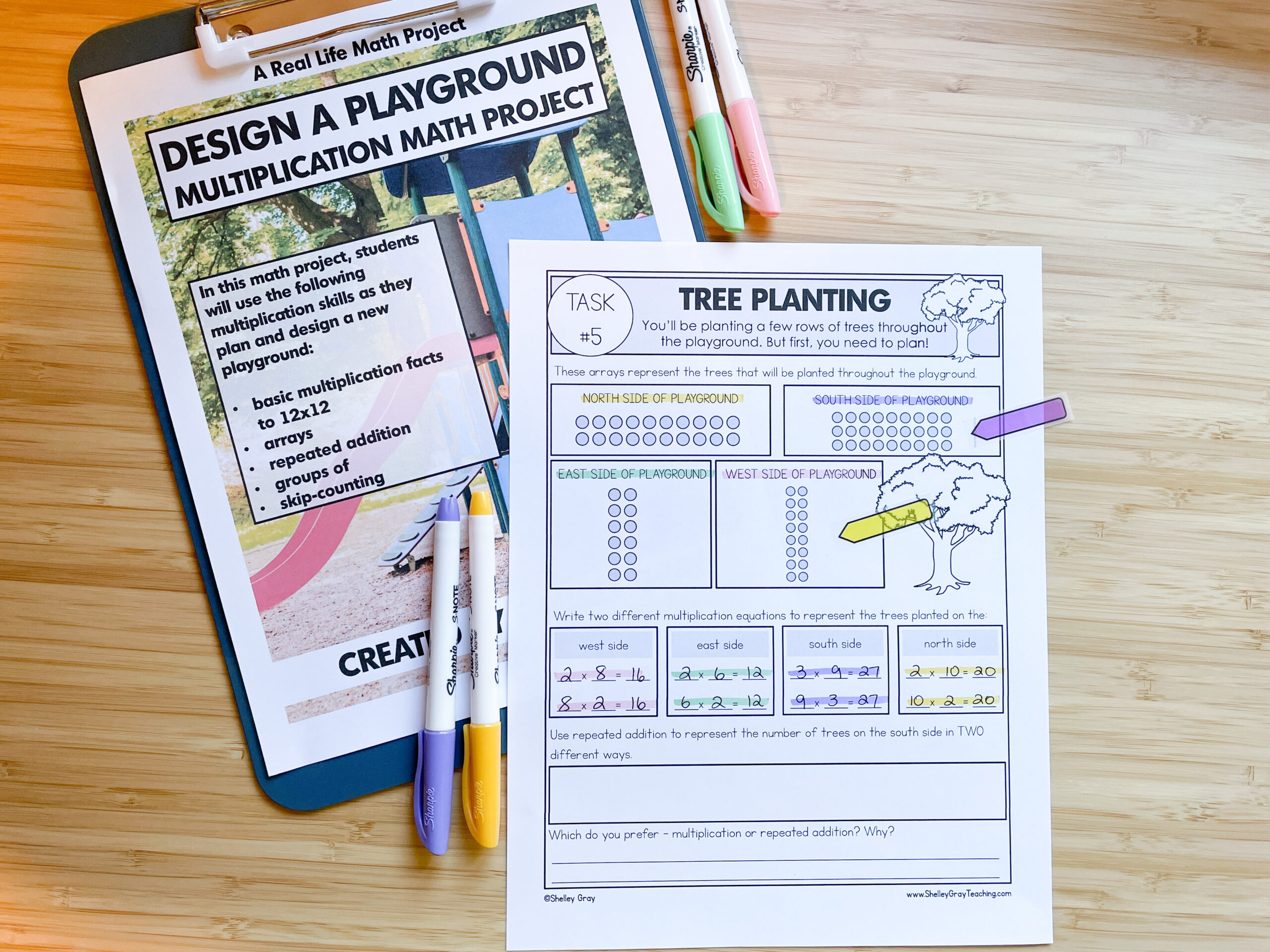Learning the multiplication facts can feel impossible and intimidating.  The result for students is often fear, lack of confidence, and a feeling of being discouraged. But what if I told you that you can help your students easily master the times tables using this one game-changing strategy? It will also make multiplication fun, interesting, and meaningful!

The one strategy that is often missing from multiplication instruction is focusing on the connections and relationships between facts.

How are different multiplication facts connected? How are they related to one another?

Understanding how the multiplication facts are connected will give kids the “a-ha” moments. This understanding is what will give kids the ability to solve ANY fact – even if it hasn’t been “memorized.” When students understand that multiplication facts are all related – that they can use one fact to help them solve another – the thought of learning the times tables suddenly becomes much less intimidating.

Here are a few simple tips for how you can help your students easily master the times tables by focusing on connections and relationships between multiplication facts.

Tip #1: Incorporate Concepts Like Doubles and Halves

Our students need to understand how facts like 3×5 and 6×5 are connected. Or how facts like 10×2 and 5×2 are connected. This concept of doubles and halves can be made visual with an array. When students can look at an array and actually SEE what the facts mean, it becomes easy to visualize 5×2 as half of 10×2.I go more into detail on this concept in this free guide – Three Reasons Your Students Aren’t Mastering The Multiplication Facts (and what you can do about it). Students must understand that multiplication facts aren’t isolated pieces of information they need to memorize in a meaningless way. Facts are related and connected, and we can use this knowledge to master the times tables…without having to memorize! When we understand connections, we can literally solve anything!Imagine how understanding doubles and halves can help when we get past basic facts? What about solving something like 35×7? We could do this quickly in our heads when we understand that it will be half of 70×7. Or how about 25×12? This understanding of doubles and halves can help us solve this by first thinking of 50×12, then simply halving the product.

Tip #2: Treat Sets of Facts as Related to One Another (Not as Isolated Pieces of Information)

When we help students see that sets of facts are related, suddenly multiplication gets a lot more fun. We don’t HAVE to memorize the facts – all we have to do is understand them!

This activity from The Multiplication Station shows how we can help students make a visual connection between the 10’s and 9’s facts. We don’t have to memorize all the 9’s facts! All we have to do is understand how they are related to the 10’s facts! It is this understanding that will enable students to easily solve a fact like 9×25 without resorting to traditional long multiplication or a calculator.

We can help students master the 9’s times tables simply by helping them understand the connection to the 10’s facts.Here’s another example, using the x2, x4, and x8 facts. Here we can see that these facts are all related. Using the first example on this page, let’s take a look at all the connections we see! 2×10 is half of 4×10. 4×10 is the double of 2×10. 4×10 is half of 8×10. The product of 8×10 is four times the product of 2×10. And it goes on and on. These connections are powerful.I encourage you to have your students work with manipulatives to help visualize these connections. Sometimes we make the mistake of thinking our students are “past” manipulatives when they get to Grade 3 or 4 but this couldn’t be further from the truth. Using objects that students can move and manipulate will help them solidify these connections and truly SEE them.Tip #3: Use Arrays

As you may have noticed, I love using arrays for multiplication. I believe that arrays are THE most powerful way to visualize multiplication. They are NOT simply for introducing multiplication. Arrays are for exploring relationships and connections between facts.

So many students don’t see arrays in their minds when they think about what multiplication means.

To help students begin visualizing arrays, we can use simple tools like a rekenrek. These pictures show how we can build an array to show 3×5 (or 3 rows of 5), and then simply rotate the rekenrek to show that 5×3 (or 5 rows of 3) has the same product (the commutative property). This is a fantastic way to make multiplication concrete.In this activity from the Design a Playground Math Project, students are using arrays in a representational way, and then connecting that to abstract thinking when they write the facts. Again, we are reinforcing the commutative property and the relationship between facts like 2×8 and 8×2.Connections have the power to change the game for your students when it comes to multiplication and learning the times tables. If you are having trouble getting started with connection-making, begin with these simple steps.

• Use manipulatives to form an array. Ask, “What can we add to this array to form a new fact?”
• Always help students make connections in the concrete and representational phase, not in the abstract. They need to SEE the connections in order to understand them. We can’t expect just to show students two written equations and have them understand the connections at a conceptual level.

The resources mentioned in this blog post are linked below:

This site uses Akismet to reduce spam. Learn how your comment data is processed.